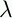September 22, 1999 Perl and the Lambda Calculus Slide #26

# Number Example

• There are many ways to make numbers. Here's a simple one

• Idea: Represent numbers with lists

```        ZERO =          (PAIR TRUE TRUE)
```
```        SUCC =n.(PAIR FALSE n)
```
```        IS_ZERO =n.(FIRST n)
```
```        PRED =n.(SECOND n)
```

 NextCopyright © 1999 M-J. Dominus